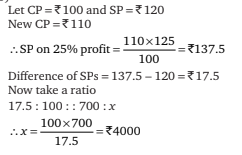# Mathematical Aptitude - Profit and Loss

>>>>>>>>Profit and Loss

• A

Rs 1,50,000• B

Rs 1,60,000• C

Rs 1,62,000• D

Rs 1,52,500• Option : C
• Explanation : Profit and loss formula is given by, Let S.P. of the car = x
Discount of 10%.
Profit = 8%.
∴ ((90/100)x – 135000/135000) × 100 = 8
⇒ (90/100)x – 135000 = 8 × 1350 ⇒ (90/100)x
⇒ x = 162000

• A

Rs 430• B

Rs 450• C

Rs 220• D

Rs 400• Option : D
• Explanation : Let C.P. of the article = 100
∴ Old S.P. = 108. New C.P. = 90. As the profit is 15%, so the new S.P. = 90 × 115/100 = 103.5. The difference in the two selling prices = 108 – 103.5 = Rs 4.5 If difference in S.P. is 4.5 then C.P. = 100. If difference in S.P. is 18, then C.P. = (100/4.5) × 18 = Rs 400.

• A

Rs 5100• B

Rs 5150• C

Rs 5400• D

Rs 5500• Option : A
• Explanation :• A

Rs 5000• B

Rs 4000• C

Rs 3800• D

None of the above• Option : B
• Explanation :• A

36%• B

38%• C

40%• D

44%• Option : D
• Explanation : SP = 90% of 8000 = Rs 7200
Profit = 7200 – 5000 = Rs 2200
Profit percentage = 2200/5000 × 100 = 44%

## Description

• Profit and Loss Problems Questions can be used to give quizzes by any candidate who is preparing for UGC NET Paper 1
• This Profit and Loss Problems Questions section will help you test your analytical skills in a tricky method, thereby giving you an edge over other students
• All candidates who have to appear for the Kendriya Vidyalaya Entrance exam can also refer to this mcq section.
• You can also get access to the Profit and Loss Problems MCQ ebook.
• Profit and Loss Problems Questions can be used in the preparation of JRF, CSIR, and various other exams.